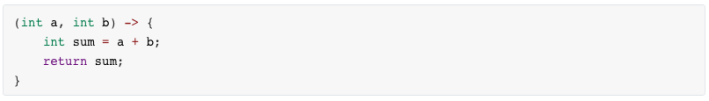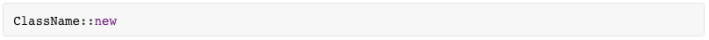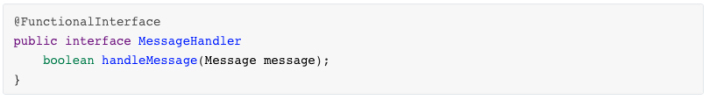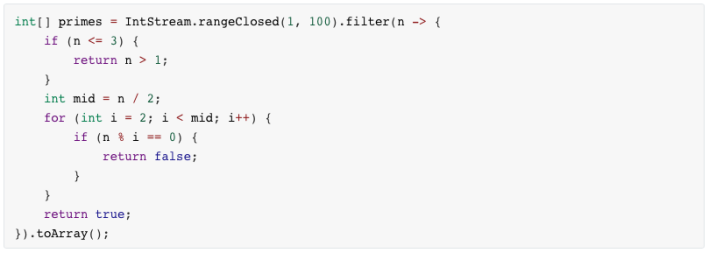# Java8 Lambda表达式教程

+关注继续查看

1. 什么是λ表达式

λ表达式本质上是一个匿名方法。让我们来看下面这个例子：

public int add(int x, int y) {
return x + y;
}

(int x, int y) -> x + y;

(x, y) -> x + y; //返回两数之和

(x, y) -> { return x + y; } //显式指明返回值

() -> { System.out.println("Hello Lambda!"); }

c -> { return c.size(); }

2. λ表达式的类型（它是Object吗？）

λ表达式可以被当做是一个Object（注意措辞）。λ表达式的类型，叫做“目标类型（target type）”。λ表达式的目标类型是“函数接口（functional interface）”，这是Java8新引入的概念。它的定义是：一个接口，如果只有一个显式声明的抽象方法，那么它就是一个函数接口。一般用@FunctionalInterface标注出来（也可以不标）。举例如下：

@FunctionalInterface
public interface Runnable { void run(); }

public interface Callable<V> { V call() throws Exception; }

public interface ActionListener { void actionPerformed(ActionEvent e); }

public interface Comparator<T> { int compare(T o1, T o2); boolean equals(Object obj); }

Runnable r1 = () -> {System.out.println("Hello Lambda!");};

Object obj = r1;

Object obj = () -> {System.out.println("Hello Lambda!");}; // ERROR! Object is not a functional interface!

Object o = (Runnable) () -> { System.out.println("hi"); }; // correct

System.out.println( () -> {} ); //错误! 目标类型不明

System.out.println( (Runnable)() -> {} ); // 正确

@FunctionalInterface
public interface MyRunnable {
public void run();
}

Runnable r1 =    () -> {System.out.println("Hello Lambda!");};
MyRunnable2 r2 = () -> {System.out.println("Hello Lambda!");};

JDK预定义了很多函数接口以避免用户重复定义。最典型的是Function：

@FunctionalInterface
public interface Function<T, R> {
R apply(T t);
}

@FunctionalInterface
public interface Consumer<T> {
void accept(T t);
}

@FunctionalInterface
public interface Predicate<T> {
boolean test(T t);
}

3. λ表达式的使用

3.1 λ表达式用在何处

@Override
public void run() {
System.out.println("This is from an anonymous class.");
}
} );

System.out.println("This is from an anonymous method (lambda exp).");
} );

3.2 λ表达式与集合类批处理操作（或者叫块操作）

Java8之前集合类的迭代（Iteration）都是外部的，即客户代码。而内部迭代意味着改由Java类库来进行迭代，而不是客户代码。例如：

for(Object o: list) { // 外部迭代
System.out.println(o);
}

list.forEach(o -> {System.out.println(o);}); //forEach函数实现内部迭代

Java8为集合类引入了另一个重要概念：流（stream）。一个流通常以一个集合类实例为其数据源，然后在其上定义各种操作。流的API设计使用了管道（pipelines）模式。对流的一次操作会返回另一个流。如同IO的API或者StringBuffer的append方法那样，从而多个不同的操作可以在一个语句里串起来。看下面的例子：

List<Shape> shapes = ...
shapes.stream()
.filter(s -> s.getColor() == BLUE)
.forEach(s -> s.setColor(RED));

filter方法的参数是Predicate类型，forEach方法的参数是Consumer类型，它们都是函数接口，所以可以使用λ表达式。

shapes.parallelStream(); // 或shapes.stream().parallel()

//给出一个String类型的数组，找出其中所有不重复的素数
public void distinctPrimary(String... numbers) {
List<String> l = Arrays.asList(numbers);
List<Integer> r = l.stream()
.map(e -> new Integer(e))
.filter(e -> Primes.isPrime(e))
.distinct()
.collect(Collectors.toList());
System.out.println("distinctPrimary result is: " + r);
}

//给出一个String类型的数组，找出其中所有不重复的素数，并统计其出现次数
public void primaryOccurrence(String... numbers) {
List<String> l = Arrays.asList(numbers);
Map<Integer, Integer> r = l.stream()
.map(e -> new Integer(e))
.filter(e -> Primes.isPrime(e))
.collect( Collectors.groupingBy(p->p, Collectors.summingInt(p->1)) );
System.out.println("primaryOccurrence result is: " + r);
}

Collectors.groupingBy(p->p, Collectors.summingInt(p->1))

//给出一个String类型的数组，求其中所有不重复素数的和
public void distinctPrimarySum(String... numbers) {
List<String> l = Arrays.asList(numbers);
int sum = l.stream()
.map(e -> new Integer(e))
.filter(e -> Primes.isPrime(e))
.distinct()
.reduce(0, (x,y) -> x+y); // equivalent to .sum()
System.out.println("distinctPrimarySum result is: " + sum);
}

reduce方法用来产生单一的一个最终结果。

// 统计年龄在25-35岁的男女人数、比例
public void boysAndGirls(List<Person> persons) {
Map<Integer, Integer> result = persons.parallelStream().filter(p -> p.getAge()>=25 && p.getAge()<=35).
collect(
Collectors.groupingBy(p->p.getSex(), Collectors.summingInt(p->1))
);
System.out.print("boysAndGirls result is " + result);
System.out.println(", ratio (male : female) is " + (float)result.get(Person.MALE)/result.get(Person.FEMALE));
}

3.3 λ表达式的更多用法

// 嵌套的λ表达式
Callable<Runnable> c1 = () -> () -> { System.out.println("Nested lambda"); };
c1.call().run();

// 用在条件表达式中
Callable<Integer> c2 = true ? (() -> 42) : (() -> 24);
System.out.println(c2.call());

// 定义一个递归函数
private UnaryOperator<Integer> factorial = i -> { return i == 0 ? 1 : i * factorial.apply( i - 1 ); };
...
System.out.println(factorial.apply(3));

int five = ( (x, y) -> x + y ) (2, 3); // ERROR! try to call a lambda in-place

4. 其它相关概念

4.1 捕获（Capture）

...
int tmp1 = 1; //包围类的成员变量
static int tmp2 = 2; //包围类的静态成员变量
public void testCapture() {
int tmp3 = 3; //没有声明为final，但是effectively final的本地变量
final int tmp4 = 4; //声明为final的本地变量
int tmp5 = 5; //普通本地变量

Function<Integer, Integer> f1 = i -> i + tmp1;
Function<Integer, Integer> f2 = i -> i + tmp2;
Function<Integer, Integer> f3 = i -> i + tmp3;
Function<Integer, Integer> f4 = i -> i + tmp4;
Function<Integer, Integer> f5 = i -> {
tmp5  += i; // 编译错！对tmp5赋值导致它不是effectively final的
return tmp5;
};
...
tmp5 = 9; // 编译错！对tmp5赋值导致它不是effectively final的
}
...

Java要求本地变量final或者effectively final的原因是多线程并发问题。内部类、λ表达式都有可能在不同的线程中执行，允许多个线程同时修改一个本地变量不符合Java的设计理念。

4.2 方法引用（Method reference）

Integer::parseInt //静态方法引用
System.out::print //实例方法引用
Person::new       //构造器引用

//c1 与 c2 是一样的（静态方法引用）
Comparator<Integer> c2 = (x, y) -> Integer.compare(x, y);
Comparator<Integer> c1 = Integer::compare;

//下面两句是一样的（实例方法引用1）
persons.forEach(e -> System.out.println(e));
persons.forEach(System.out::println);

//下面两句是一样的（实例方法引用2）
persons.forEach(person -> person.eat());
persons.forEach(Person::eat);

//下面两句是一样的（构造器引用）
strList.stream().map(s -> new Integer(s));
strList.stream().map(Integer::new);

public void distinctPrimarySum(String... numbers) {
List<String> l = Arrays.asList(numbers);
int sum = l.stream().map(Integer::new).filter(Primes::isPrime).distinct().sum();
System.out.println("distinctPrimarySum result is: " + sum);
}

super::methName //引用某个对象的父类方法
TypeName[]::new //引用一个数组的构造器

4.3 默认方法（Default method）

Java8中，接口声明里可以有方法实现了，叫做默认方法。在此之前，接口里的方法全部是抽象方法。

public interface MyInterf {

String m1();

default String m2() {
return "Hello default method!";
}

}

public class Sub implements Base1, Base2 {

public void hello() {
Base1.super.hello(); //使用Base1的实现
}

}

public interface MyInterf {

String m1();

default String m2() {
return "Hello default method!";
}

static String m3() {
return "Hello static method in Interface!";
}

}

4.4 生成器函数（Generator function）

Stream.generate(Math::random).limit(5).forEach(System.out::println);《Java工程师必读手册》——Java极客主义系列——最完美的Lambda表达式只有一行（1）
《Java工程师必读手册》——Java极客主义系列——最完美的Lambda表达式只有一行（1）
16 0《Java工程师必读手册》——Java极客主义系列——最完美的Lambda表达式只有一行（2）
《Java工程师必读手册》——Java极客主义系列——最完美的Lambda表达式只有一行（2）
24 0《Java工程师必读手册》——Java极客主义系列——最完美的Lambda表达式只有一行（3）
《Java工程师必读手册》——Java极客主义系列——最完美的Lambda表达式只有一行（3）
18 0《Java工程师必读手册》——Java极客主义系列——最完美的Lambda表达式只有一行（4）
《Java工程师必读手册》——Java极客主义系列——最完美的Lambda表达式只有一行（4）
16 0《Java工程师必读手册》——Java极客主义系列——最完美的Lambda表达式只有一行（5）
《Java工程师必读手册》——Java极客主义系列——最完美的Lambda表达式只有一行（5）
12 0

Java编程入门1489830

JAVA开发手册1.5.0